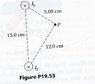# Straight wired magnetism

• cy19861126

## Homework Statement

Two long parallel conductors carry currents I1 = 3.00A and I2 = 3.00 A, both directed into the page as shown below. Determine the magnitude and direction of the resultant magnetic field at P.

## Homework Equations

B = uI/(2*3.14*r)
u = 1.26*10^-6 T*m/A

## The Attempt at a Solution

B(I1) = 1.26*10^-6 * 3.00/(2*3.14*0.05) = 1.2*10^-5T
B(I2) = 1.26*10^-6 * 3.00/(2*3.14*0.12) = 5*10^-6T
Now I am having trouble figuring out which direction it is going. Any help would be good. Thanks! I am familiar with the right hand rule, where if you point the thumb to where the current is going and curl the finger, the finger points toward the direction of the magnetic field. The answer for this question is 13.0uT toward the bottom of the page

#### Attachments

•#53.JPG
7.9 KB · Views: 907
Last edited:
So, how about the field from I1, do you know which way it points at P?